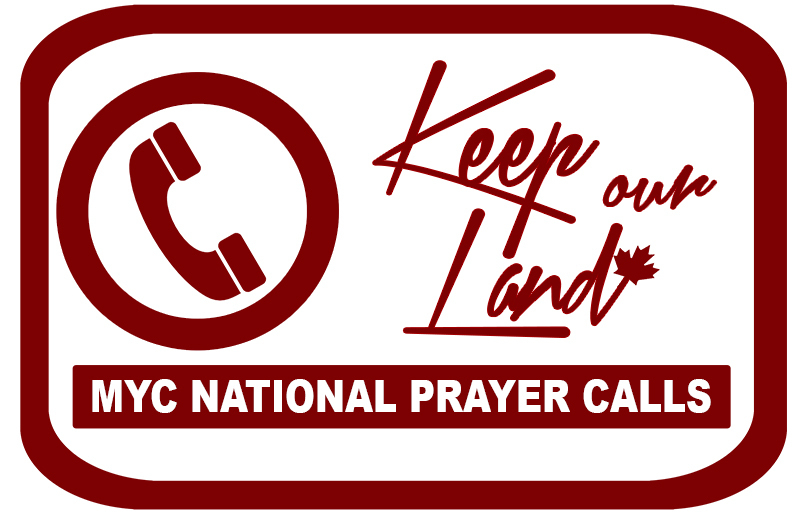Like   Tweet   Pin   +1   in
 /* styles */ December 7th, 2017

Brad Wall has been a highly popular Premier throughout his ten years in leadership, having won the third-largest majority in SK's history in 2011 and 51 of the 61 seats in the legislature in 2016.

Especially since the new party leader will also become the province's next Premier, this election provides a significant opportunity to elect a righteous leader to this important position in the province and in the nation. We believe that all of our members who live in SK should be a part of making the decision!

## Act Now To Get Your Vote:

In order to vote in the January 27th election, you must sign up for a membership by the December 8th deadline! That means, if you live in SK and do not currently have a membership to the Saskatchewan Party, you should click here now to get one!

Memberships cost \$10 each and you only need to be 14 years old to buy one and participate in this significant election.

(If you already have a membership, please be sure that it is paid up to the voting dates so that your vote is counted.)

## Right Now's Suggested Ballot:

Right Now has created a document outlining their suggestions for the ballot based on interviews with the different candidates on issues surrounding abortion and euthanasia. We encourage you to do your own prayer and research when filling out your ballot but this report is an excellent starting point and suggestion.

Click here to view Right Now's ranked ballot report including interviews with the candidates.

 table div table+table+table+table+table div table{width:100%;padding:0}table div table+table+table+table+table div table img{width:96.23%;padding:0;float:none}table div table+table+table+table+table div table td{width:100%;padding:0 1.88% 18px}/* styles */## VOLUNTEERS NEEDED: December 11th By-elections

 table div table+table+table+table+table+table+table div table td,table.module-6{width:100%;padding:0}table div table+table+table+table+table+table+table div table{width:100%;float:none;margin-left:auto;margin-right:auto;padding:0}table div table+table+table+table+table+table+table div table a{border:0 none;text-decoration:none}table div table+table+table+table+table+table+table div table img{width:100%!important;border:0 none;text-decoration:none}/* styles */
 /* styles */ We are right around the corner from a number of other important electoral opportunities beginning with the federal by-elections happening this coming Monday, December 11th. MY Canada is working with a number of godly candidates and their campaigns to ensure that they win their elections. But we need your help to be successful. If you can give a few hours of your time this weekend or on December 11th to helping godly candidates, please let us know You are especially needed if you live in Saskatchewan or in the Greater Toronto Area. Please e-mail us now at admin@4mycanada.ca if you are able to help.
 table div table+table+table+table+table+table+table+table+table div table{width:100%;padding:0}table div table+table+table+table+table+table+table+table+table div table img{width:96.23%;padding:0;float:none}table div table+table+table+table+table+table+table+table+table div table td{width:100%;padding:0 1.88% 18px}/* styles */table.module-9{width:98.49%;padding:0}table div table+table+table+table+table+table+table+table+table+table div table{width:98.49%;float:none;margin-left:auto;margin-right:auto;padding:0}table div table+table+table+table+table+table+table+table+table+table div table a{border:0 none;text-decoration:none}table div table+table+table+table+table+table+table+table+table+table div table img{width:100%!important;border:0 none;text-decoration:none}table div table+table+table+table+table+table+table+table+table+table div table td{width:100%;padding:0}/* styles */
 table div table+table+table+table+table+table+table+table+table+table+table div table{width:100%;padding:0}table div table+table+table+table+table+table+table+table+table+table+table div table img{width:96.23%;padding:0;float:none}table div table+table+table+table+table+table+table+table+table+table+table div table td{width:100%;padding:0 1.88% 18px}/* styles */## ANNOUNCEMENTS:

 table div table+table+table+table+table+table+table+table+table+table+table+table+table div table{width:100%;padding:0}table div table+table+table+table+table+table+table+table+table+table+table+table+table div table img{width:96.23%;padding:0;float:none}table div table+table+table+table+table+table+table+table+table+table+table+table+table div table td{width:100%;padding:0 1.88% 18px}/* styles */## Interested in Running for Election?

We can help! MY Canada is currently conducting a series of trainings and webinars to help godly candidates get started in their campaigns for office. Please e-mail admin@4mycanada.ca if you are interested in learning more.

 table div table+table+table+table+table+table+table+table+table+table+table+table+table+table+table div table{width:100%;padding:0}table div table+table+table+table+table+table+table+table+table+table+table+table+table+table+table div table img{width:96.23%;padding:0;float:none}table div table+table+table+table+table+table+table+table+table+table+table+table+table+table+table div table td{width:100%;padding:0 1.88% 18px}/* styles */table.module-15{width:99.81%;padding:0}table div table+table+table+table+table+table+table+table+table+table+table+table+table+table+table+table div table{width:99.81%;float:none;margin-left:auto;margin-right:auto;padding:0}table div table+table+table+table+table+table+table+table+table+table+table+table+table+table+table+table div table a{border:0 none;text-decoration:none}table div table+table+table+table+table+table+table+table+table+table+table+table+table+table+table+table div table img{width:100%!important;border:0 none;text-decoration:none}table div table+table+table+table+table+table+table+table+table+table+table+table+table+table+table+table div table td{width:100%;padding:0}/* styles */
 /* styles */ The new Justice Wall website is now live and allows for many thousands of individuals to sign up to pray for the ending of abortion and human trafficking and for the persecuted Church around the world. Will you help us build a 24-7 virtual prayer wall for these issues? Click here or visit www.JusticeWall.com to sign up for your 15-minute prayer slot now.
 table div table+table+table+table+table+table+table+table+table+table+table+table+table+table+table+table+table+table div table{width:100%;padding:0}table div table+table+table+table+table+table+table+table+table+table+table+table+table+table+table+table+table+table div table img{width:96.23%;padding:0;float:none}table div table+table+table+table+table+table+table+table+table+table+table+table+table+table+table+table+table+table div table td{width:100%;padding:0 1.88% 18px}/* styles */table div table+table+table+table+table+table+table+table+table+table+table+table+table+table+table+table+table+table+table+table div table{width:100%;padding:0}table div table+table+table+table+table+table+table+table+table+table+table+table+table+table+table+table+table+table+table+table div table img{width:96.23%;padding:0;float:none}table div table+table+table+table+table+table+table+table+table+table+table+table+table+table+table+table+table+table+table+table div table td{width:100%;padding:0 1.88% 18px}/* styles */MY Canada is hosting national prayer conference calls every second Tuesday at noon EST.

The next call is December 19th, 2017.

 table div table+table+table+table+table+table+table+table+table+table+table+table+table+table+table+table+table+table+table+table+table+table div table{width:100%;padding:0}table div table+table+table+table+table+table+table+table+table+table+table+table+table+table+table+table+table+table+table+table+table+table div table img{width:96.23%;padding:0;float:none}table div table+table+table+table+table+table+table+table+table+table+table+table+table+table+table+table+table+table+table+table+table+table div table td{width:100%;padding:0 1.88% 18px}/* styles */table div table+table+table+table+table+table+table+table+table+table+table+table+table+table+table+table+table+table+table+table+table+table+table+table div table{width:100%;padding:0}table div table+table+table+table+table+table+table+table+table+table+table+table+table+table+table+table+table+table+table+table+table+table+table+table div table img{width:96.23%;padding:0;float:none}table div table+table+table+table+table+table+table+table+table+table+table+table+table+table+table+table+table+table+table+table+table+table+table+table div table td{width:100%;padding:0 1.88% 18px}/* styles */